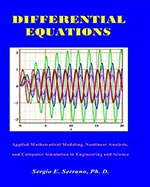Differential Equations. Applied Mathematical Modeling, Nonlinear Analysis, and Computer Simulation in Engineering and Science. - Maplesoft Books - Maple Books, Maple Resources and Math BooksHome : Maplesoft Books : Differential Equations. Applied Mathematical Modeling, Nonlinear Analysis, and Computer Simulation in Engineering and Science.Details Search# Differential Equations. Applied Mathematical Modeling, Nonlinear Analysis, and Computer Simulation in Engineering and Science.

## 2016

Description

An introduction to ordinary differential equations and systems of ordinary differential equations, including new analytical methods to solve nonlinear equations, mathematical modeling, computer programming, computer graphics with Maple, and applications in science and engineering.

New modern methods to solve nonlinear differential equations.

• Integrated, balanced presentation of model development, solution methods, computer implementation, and analysis of system’s response.
• Designed to increase engineering and science student retention.
• Mathematical and computer modeling with Maple. No prior knowledge of MAPLE required.
• Emphasis on problem solving over mathematical rigorousness.
• Clear and simple presentation of concepts with multitude of examples: 125 solved examples, 70 MAPLE programs, 146 proposed problems, 17 illustrations, 118 computer graphs, answers to problems, detailed bibliography.
• Preference of general, systematic, analytical methods over complex numerical methods.
• Self contained. Except for calculus, all necessary background is included.

•

Categories:
Other Details
Language: English
ISBN: 978-0988865211
Publisher: HydroScience Inc.
 Do you know of a book that should be included in our list? Tell us about it!
 Title/Author: Category: Any Category Abstract Algebra Biology Calculus Chemistry Complex Analysis Computer Algebra Computer Science Conference Proceedings Courseware Cryptography Differential Equations Differential Geometry Dynamical Systems Economics Engineering Games Linear Algebra Maple Manuals Mathematical Modeling Number Theory Numerical Analysis Operations Research Optimization Physics Pre-Calculus Probability & Statistics Programming Recent Releases Vector Calculus Language: Any Language English French German Spanish Japanese Dutch Russian Swedish Polish Persian ChineseAbstract Algebra Biology Calculus Chemistry Complex Analysis Computer Algebra Computer Science Conference Proceedings Courseware Cryptography Differential Equations Differential Geometry Dynamical Systems Economics Engineering Games Linear Algebra Maple Manuals Mathematical Modeling Number Theory Numerical Analysis Operations Research Optimization Physics Pre-Calculus Probability & Statistics Programming Recent Releases Vector Calculus